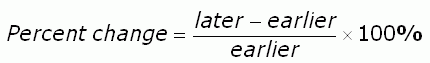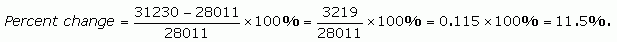SEARCH HOMEMath Central Quandaries & QueriesQuestion from Galland: I am working on graphs and I need to find the percentage of increase from one figure to another. How do I do that? For instance staff hours for 2006 were 28011 and for 2007 were 31230. How do I find the percentage of increase?Hi Galland.

Percentage change is calculated this way:If both later and earlier are positive then the sign of the percentage change indicates whether it is a decrease or an increase. For your example,Since this is a positive figure, it is an 11.5% increase.

If one of later or earlier is negative we suggest you look at RD's response to such a question.

Hope this helps,
Stephen La Rocque.Math Central is supported by the University of Regina and The Pacific Institute for the Mathematical Sciences.